Courses

# First Law Of Thermodynamics NAT Level – 2

## 10 Questions MCQ Test Topic wise Tests for IIT JAM Physics | First Law Of Thermodynamics NAT Level – 2

Description
This mock test of First Law Of Thermodynamics NAT Level – 2 for IIT JAM helps you for every IIT JAM entrance exam. This contains 10 Multiple Choice Questions for IIT JAM First Law Of Thermodynamics NAT Level – 2 (mcq) to study with solutions a complete question bank. The solved questions answers in this First Law Of Thermodynamics NAT Level – 2 quiz give you a good mix of easy questions and tough questions. IIT JAM students definitely take this First Law Of Thermodynamics NAT Level – 2 exercise for a better result in the exam. You can find other First Law Of Thermodynamics NAT Level – 2 extra questions, long questions & short questions for IIT JAM on EduRev as well by searching above.
*Answer can only contain numeric values
QUESTION: 1

### 5000J of heat are added to two moles an ideal monoatomic gas, initially at a temperature of 500K, while the gas performs 7500J of work. What is the final temperature of the gas ? (in Kelvin)

Solution:

ΔU = ΔQ – ΔW = 5000 - 7500
ΔU = –2500 J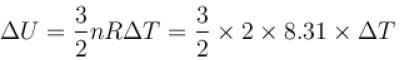⇒ ΔT = –100 K
⇒ T = 500 - 100 = 400 K

*Answer can only contain numeric values
QUESTION: 2

### For a diatomic ideal gas near room temperature, what fraction of heat supplied is available for external work if gas is expanded at constant pressure

Solution:

In the process of expansion at constant pressure p, assuming that the volume increases from V1 to V2 and temperature change from T1 to T2 , we have
pV1 = nRT1
pV2 = nRT2
In this process, the work done by the system on the outside world is W = p(V2 -V1 )= nRΔT and increase in internal energy of the system is
ΔU = CvΔT
∴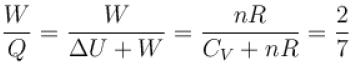*Answer can only contain numeric values
QUESTION: 3

### A gas has pressure p and volume V. It is now compressed adiabatically to 1/32 times the original volume. If (32)1.4 = 128, the final pressure in terms of p is

Solution: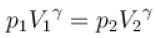⇒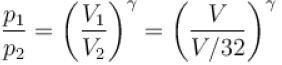⇒ p = (32)1.4 p
⇒ p1 = 128p.

*Answer can only contain numeric values
QUESTION: 4

1cm3 of water at its boiling point absorbs 540 calories of heat to become steam with a volume of 1671 cm3. If the atmospheric pressure is 1.013 × 105 N/m2 and mechanical equivalent of heat = 4.19 J/calorie, the energy spent in this process in overcoming intermolecular forces is (in calories)

Solution:

Energy spent in overcoming intermolecular forces
ΔU = ΔQ – ΔW
= ΔQ – p(V2 – V1)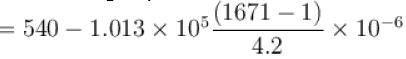= 500cal.

*Answer can only contain numeric values
QUESTION: 5

An ideal gas at 27ºC is compressed adiabatically to 8/27 of its original volume. If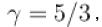then the rise in temperature (in Kelvin) is

Solution:

For adiabatic process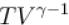= constant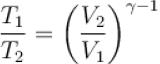⇒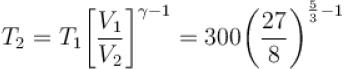T2 = 675K
ΔT =  675–300
= 375K

*Answer can only contain numeric values
QUESTION: 6

Two systems with heat capacities 100cal/gm-K and 200cal/gm-K interact thermally and come to a common temperature 400K. If the initial temperature of system 1 is 500K, what was the initial temperature of systems (in Kelvin) scale

Solution:

Let initial temperature of system 2 is T2. According to conservation of energy, we know that heat released from one system is equal to the heat absorbed by the other system, i.e.
C1 (Tf – T1 ) = C2 (T2 –Tf )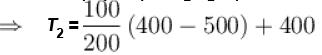= –50+400
T2 = 350K

*Answer can only contain numeric values
QUESTION: 7

During an adiabatic processes, the pressure of a gas is found to be proportional to the cube of its absolute temperature. The ratio Cp /CV for the gas is

Solution: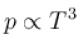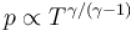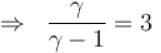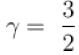⇒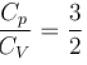*Answer can only contain numeric values
QUESTION: 8

When an ideal gas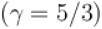is heated under constant pressure, then what percentage of given heat energy will be utilized in doing external work?

Solution: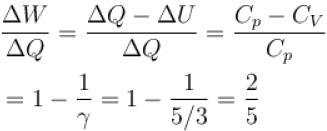∴ Percentage energy utilized in doing external work =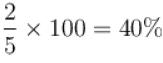*Answer can only contain numeric values
QUESTION: 9

5 mole of hydrogen gas is heated from 30ºC to 60ºC at constant pressure. Heat given to the gas is in calories (given R = 2cal/mole-ºC)

Solution: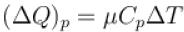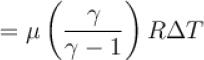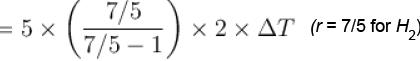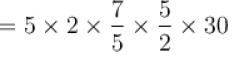= 1050 calories

*Answer can only contain numeric values
QUESTION: 10

Three liquids with masses m1, m2, m3 are thoroughly mixed. If their specific heats are C1, C2 and C3 and their temperature T1, T2, T3, then the temperature of the mixture is given that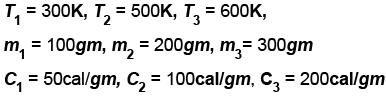Solution:

Let the final temperature be T K. Total heat supplied by 3 liquids in coming down to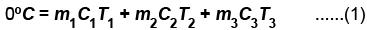Total heat used by three liquids in raising temperature from 0º to TºC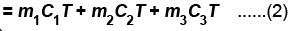from (1) & (2),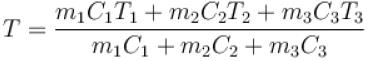⇒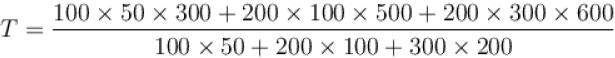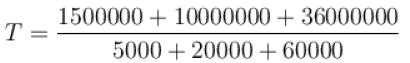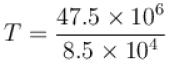T = 558.8K

Track your progress, build streaks, highlight & save important lessons and more!

### Similar Content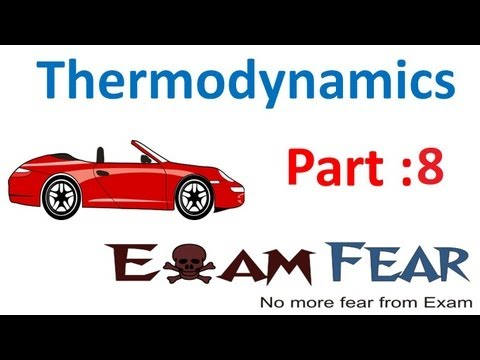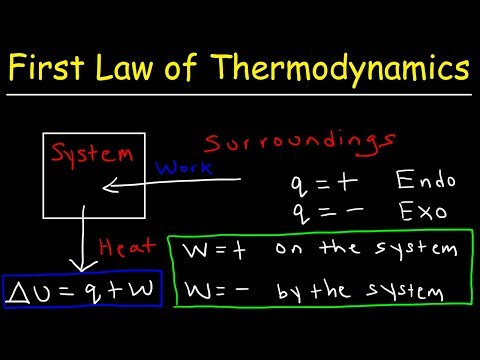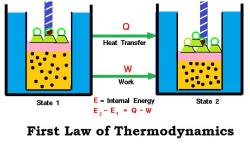### Related tests